## 9.5 Collisions in Multiple Dimensions

### Learning Objectives

By the end of this section, you will be able to:

• Express momentum as a two-dimensional vector
• Write equations for momentum conservation in component form
• Calculate momentum in two dimensions, as a vector quantity

It is far more common for collisions to occur in two dimensions; that is, the angle between the initial velocity vectors is neither zero nor $180\text{°}$. Let’s see what complications arise from this.

The first idea we need is that momentum is a vector; like all vectors, it can be expressed as a sum of perpendicular components (usually, though not always, an x-component and a y-component, and a z-component if necessary). Thus, when we write down the statement of conservation of momentum for a problem, our momentum vectors can be, and usually will be, expressed in component form.

The second idea we need comes from the fact that momentum is related to force:

$\overset{\to }{F}=\frac{d\overset{\to }{p}}{dt}.$

Expressing both the force and the momentum in component form,

${F}_{x}=\frac{d{p}_{x}}{dt},\enspace{F}_{y}=\frac{d{p}_{y}}{dt},\enspace{F}_{z}=\frac{d{p}_{z}}{dt}.$

Remember, these equations are simply Newton’s second law, in vector form and in component form. We know that Newton’s second law is true in each direction, independently of the others. It follows therefore (via Newton’s third law) that conservation of momentum is also true in each direction independently.

These two ideas motivate the solution to two-dimensional problems: We write down the expression for conservation of momentum twice: once in the x-direction and once in the y-direction.

$\begin{array}{ccc}\hfill {p}_{\text{f},x}& =\hfill & {p}_{\text{1,i},x}+{p}_{\text{2,i},x}\hfill \\ \hfill {p}_{\text{f},y}& =\hfill & {p}_{\text{1,i},y}+{p}_{\text{2,i},y}\hfill \end{array}$

This procedure is shown graphically in (Figure).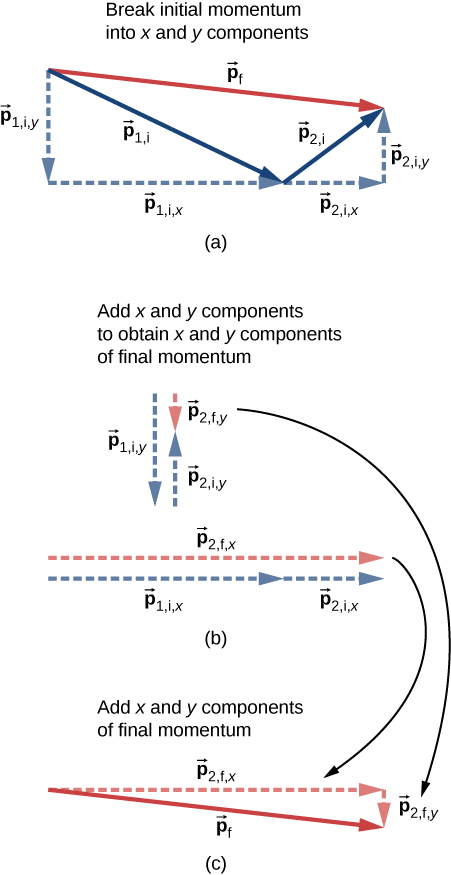Figure 9.22 (a) For two-dimensional momentum problems, break the initial momentum vectors into their x- and y-components. (b) Add the x- and y-components together separately. This gives you the x- and y-components of the final momentum, which are shown as red dashed vectors. (c) Adding these components together gives the final momentum.

We solve each of these two component equations independently to obtain the x– and y-components of the desired velocity vector:

$\begin{array}{ccc}\hfill {v}_{\text{f},x}& =\hfill & \frac{{m}_{1}{v}_{\text{1,i},x}+{m}_{2}{v}_{\text{2,i},x}}{m}\hfill \\ \hfill {v}_{\text{f},y}& =\hfill & \frac{{m}_{1}{v}_{\text{1,i},y}+{m}_{2}{v}_{\text{2,i},y}}{m}.\hfill \end{array}$

(Here, m represents the total mass of the system.) Finally, combine these components using the Pythagorean theorem,

${v}_{\text{f}}=|{\overset{\to }{v}}_{f}|=\sqrt{{v}_{\text{f},x}^{2}+{v}_{\text{f},y}^{2}}.$

### Problem-Solving Strategy: Conservation of Momentum in Two Dimensions

The method for solving a two-dimensional (or even three-dimensional) conservation of momentum problem is generally the same as the method for solving a one-dimensional problem, except that you have to conserve momentum in both (or all three) dimensions simultaneously:

1. Identify a closed system.
2. Write down the equation that represents conservation of momentum in the x-direction, and solve it for the desired quantity. If you are calculating a vector quantity (velocity, usually), this will give you the x-component of the vector.
3. Write down the equation that represents conservation of momentum in the y-direction, and solve. This will give you the y-component of your vector quantity.
4. Assuming you are calculating a vector quantity, use the Pythagorean theorem to calculate its magnitude, using the results of steps 3 and 4.

### Example

#### Traffic Collision

A small car of mass 1200 kg traveling east at 60 km/hr collides at an intersection with a truck of mass 3000 kg that is traveling due north at 40 km/hr ((Figure)). The two vehicles are locked together. What is the velocity of the combined wreckage?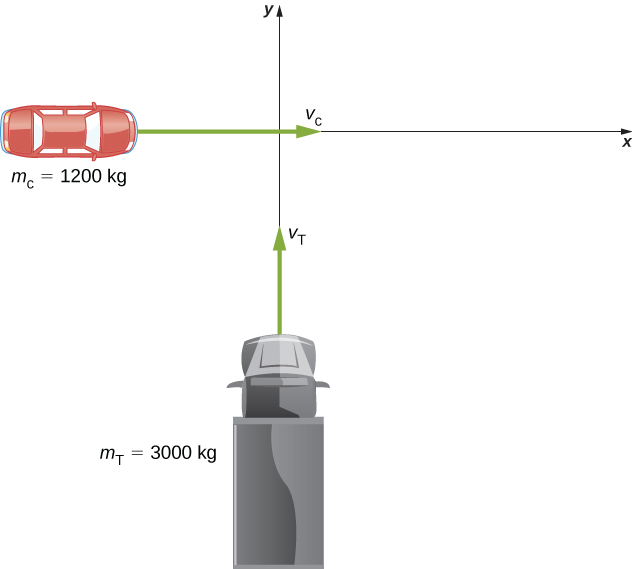Figure 9.23 A large truck moving north is about to collide with a small car moving east. The final momentum vector has both x- and y-components.

#### Strategy

First off, we need a closed system. The natural system to choose is the (car + truck), but this system is not closed; friction from the road acts on both vehicles. We avoid this problem by restricting the question to finding the velocity at the instant just after the collision, so that friction has not yet had any effect on the system. With that restriction, momentum is conserved for this system.

Since there are two directions involved, we do conservation of momentum twice: once in the x-direction and once in the y-direction.

SolutionBefore the collision the total momentum is

$\overset{\to }{p}={m}_{\text{c}}{\overset{\to }{v}}_{\text{c}}+{m}_{\text{T}}{\overset{\to }{v}}_{\text{T}}.$

After the collision, the wreckage has momentum

$\overset{\to }{p}=({m}_{\text{c}}+{m}_{\text{T}}){\overset{\to }{v}}_{w}.$

Since the system is closed, momentum must be conserved, so we have

${m}_{\text{c}}{\overset{\to }{v}}_{c}+{m}_{\text{T}}{\overset{\to }{v}}_{\text{T}}=({m}_{\text{c}}+{m}_{\text{T}}){\overset{\to }{v}}_{w}.$

We have to be careful; the two initial momenta are not parallel. We must add vectorially ((Figure)).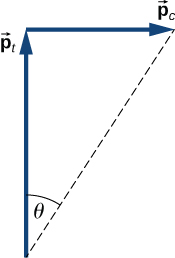Figure 9.24 Graphical addition of momentum vectors. Notice that, although the car’s velocity is larger than the truck’s, its momentum is smaller.

If we define the +x-direction to point east and the +y-direction to point north, as in the figure, then (conveniently),

$\begin{array}{ccc}\hfill {\overset{\to }{p}}_{\text{c}}& =\hfill & {p}_{\text{c}}\hat{i}={m}_{\text{c}}{v}_{\text{c}}\hat{i}\hfill \\ \hfill {\overset{\to }{p}}_{\text{T}}& =\hfill & {p}_{\text{T}}\hat{j}={m}_{\text{T}}{v}_{\text{T}}\hat{j}.\hfill \end{array}$

Therefore, in the x-direction:

$\begin{array}{ccc}\hfill {m}_{\text{c}}{v}_{\text{c}}& =\hfill & ({m}_{\text{c}}+{m}_{\text{T}}){v}_{\text{w},x}\hfill \\ \hfill {v}_{\text{w},x}& =\hfill & (\frac{{m}_{\text{c}}}{{m}_{\text{c}}+{m}_{\text{T}}}){v}_{\text{c}}\hfill \end{array}$

and in the y-direction:

$\begin{array}{ccc}\hfill {m}_{\text{T}}{v}_{\text{T}}& =\hfill & ({m}_{\text{c}}+{m}_{\text{T}}){v}_{\text{w},y}\hfill \\ \hfill {v}_{\text{w},y}& =\hfill & (\frac{{m}_{\text{T}}}{{m}_{\text{c}}+{m}_{\text{T}}}){v}_{\text{T}}.\hfill \end{array}$

Applying the Pythagorean theorem gives

$\begin{array}{cc}\hfill |{\overset{\to }{v}}_{w}|& =\sqrt{{[(\frac{{m}_{\text{c}}}{{m}_{\text{c}}+{m}_{t}}){v}_{\text{c}}]}^{2}+{[(\frac{{m}_{t}}{{m}_{\text{c}}+{m}_{t}}){v}_{t}]}^{2}}\hfill \\ & =\sqrt{{[(\frac{1200\,\text{kg}}{4200\,\text{kg}})(16.67\,\frac{\text{m}}{\text{s}})]}^{2}+{[(\frac{3000\,\text{kg}}{4200\,\text{kg}})(11.1\,\frac{\text{m}}{\text{s}})]}^{2}}\hfill \\ & =\sqrt{{(4.76\,\frac{\text{m}}{\text{s}})}^{2}+{(7.93\,\frac{\text{m}}{\text{s}})}^{2}}\hfill \\ & =9.25\,\frac{\text{m}}{\text{s}}\approx 33.3\,\frac{\text{km}}{\text{hr}}.\hfill \end{array}$

As for its direction, using the angle shown in the figure,

$\theta ={\text{tan}}^{-1}(\frac{{v}_{\text{w},x}}{{v}_{\text{w},y}})={\text{tan}}^{-1}(\frac{7.93\,\text{m/s}}{4.76\,\text{m/s}})=59\text{°}.$

This angle is east of north, or $31\text{°}$ counterclockwise from the +x-direction.

#### Significance

As a practical matter, accident investigators usually work in the “opposite direction”; they measure the distance of skid marks on the road (which gives the stopping distance) and use the work-energy theorem along with conservation of momentum to determine the speeds and directions of the cars prior to the collision. We saw that analysis in an earlier section.

Suppose the initial velocities were not at right angles to each other. How would this change both the physical result and the mathematical analysis of the collision?

### Example

#### Exploding Scuba Tank

A common scuba tank is an aluminum cylinder that weighs 31.7 pounds empty ((Figure)). When full of compressed air, the internal pressure is between 2500 and 3000 psi (pounds per square inch). Suppose such a tank, which had been sitting motionless, suddenly explodes into three pieces. The first piece, weighing 10 pounds, shoots off horizontally at 235 miles per hour; the second piece (7 pounds) shoots off at 172 miles per hour, also in the horizontal plane, but at a $19\text{°}$ angle to the first piece. What is the mass and initial velocity of the third piece? (Do all work, and express your final answer, in SI units.)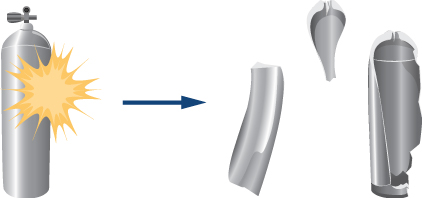Figure 9.25 A scuba tank explodes into three pieces.

#### Strategy

To use conservation of momentum, we need a closed system. If we define the system to be the scuba tank, this is not a closed system, since gravity is an external force. However, the problem asks for the just the initial velocity of the third piece, so we can neglect the effect of gravity and consider the tank by itself as a closed system. Notice that, for this system, the initial momentum vector is zero.

We choose a coordinate system where all the motion happens in the xy-plane. We then write down the equations for conservation of momentum in each direction, thus obtaining the x– and y-components of the momentum of the third piece, from which we obtain its magnitude (via the Pythagorean theorem) and its direction. Finally, dividing this momentum by the mass of the third piece gives us the velocity.

#### Solution

First, let’s get all the conversions to SI units out of the way:

$\begin{array}{cc} 31.7\,\text{lb}\,×\,\frac{1\text{\hspace{0.17em}}\text{kg}}{2.2\,\text{lb}}\to 14.4\,\text{kg}\hfill \\ 10\,\text{lb}\to 4.5\,\text{kg}\hfill \\ 235\,\frac{\text{miles}}{\text{hour}}\,×\,\frac{1\,\text{hour}}{3600\,\text{s}}\,×\,\frac{1609\,\text{m}}{\text{mile}}=105\,\frac{\text{m}}{\text{s}}\hfill \\ 7\,\text{lb}\to 3.2\,\text{kg}\hfill \\ 172\,\frac{\text{mile}}{\text{hour}}=77\,\frac{\text{m}}{\text{s}}\hfill \\ {m}_{3}=14.4\,\text{kg}-(4.5\,\text{kg}+3.2\,\text{kg})=6.7\,\text{kg.}\hfill \end{array}$

Now apply conservation of momentum in each direction.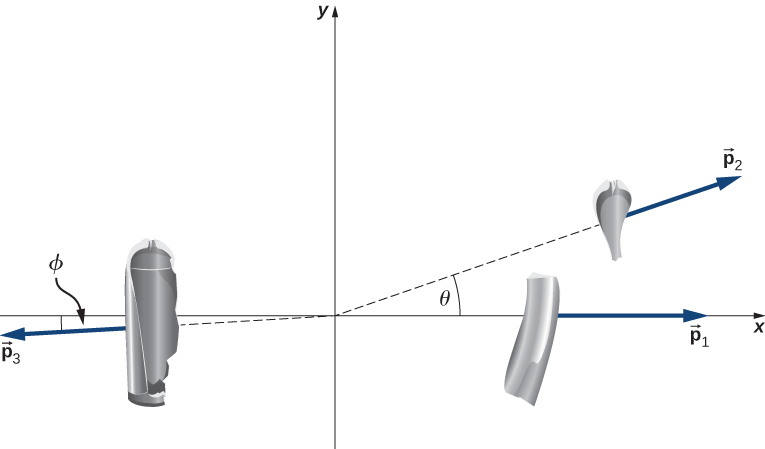x-direction:

$\begin{array}{ccc}\hfill {p}_{\text{f,}x}& =\hfill & {p}_{\text{0,}x}\hfill \\ \hfill {p}_{\text{1,}x}+{p}_{\text{2,}x}+{p}_{\text{3,}x}& =\hfill & 0\hfill \\ \hfill {m}_{1}{v}_{\text{1,}x}+{m}_{2}{v}_{\text{2,}x}+{p}_{\text{3,}x}& =\hfill & 0\hfill \\ \hfill {p}_{\text{3,}x}& =\hfill & \text{−}{m}_{1}{v}_{\text{1,}x}-{m}_{2}{v}_{\text{2,}x}\hfill \end{array}$

y-direction:

$\begin{array}{ccc}\hfill {p}_{\text{f,}y}& =\hfill & {p}_{\text{0,}y}\hfill \\ \hfill {p}_{\text{1,}y}+{p}_{\text{2,}y}+{p}_{\text{3,}y}& =\hfill & 0\hfill \\ \hfill {m}_{1}{v}_{\text{1,}y}+{m}_{2}{v}_{\text{2,}y}+{p}_{\text{3,}y}& =\hfill & 0\hfill \\ \hfill {p}_{\text{3,}y}& =\hfill & \text{−}{m}_{1}{v}_{\text{1,}y}-{m}_{2}{v}_{\text{2,}y}\hfill \end{array}$

From our chosen coordinate system, we write the x-components as

$\begin{array}{cc}\hfill {p}_{3,x}& =\text{−}{m}_{1}{v}_{1}-{m}_{2}{v}_{2}\text{cos}\theta \hfill \\ & =\text{−}(14.5\,\text{kg})(105\,\frac{\text{m}}{\text{s}})-(4.5\,\text{kg})(77\,\frac{\text{m}}{\text{s}})\text{cos}(19\text{°})\hfill \\ & =-1850\frac{\text{kg}·\text{m}}{\text{s}}.\hfill \end{array}$

For the y-direction, we have

$\begin{array}{cc}\hfill {p}_{3y}& =0-{m}_{2}{v}_{2}\text{sin}\theta \hfill \\ & =\text{−}(4.5\,\text{kg})(77\,\frac{\text{m}}{\text{s}})\text{sin}(19\text{°})\hfill \\ & =-113\,\frac{\text{kg}·\text{m}}{\text{s}}.\hfill \end{array}$

This gives the magnitude of ${p}_{3}$:

$\begin{array}{cc}\hfill {p}_{3}& =\sqrt{{p}_{\text{3,}x}^{2}+{p}_{\text{3,}y}^{2}}\hfill \\ & =\sqrt{{(-1850\,\frac{\text{kg}·\text{m}}{\text{s}})}^{2}+(-113\,\frac{\text{kg}·\text{m}}{\text{s}})}\hfill \\ & =1854\,\frac{\text{kg}·\text{m}}{\text{s}}.\hfill \end{array}$

The velocity of the third piece is therefore

${v}_{3}=\frac{{p}_{3}}{{m}_{3}}=\frac{1854\,\frac{\text{kg}·\text{m}}{\text{s}}}{6.7\,\text{kg}}=277\,\frac{\text{m}}{\text{s}}.$

The direction of its velocity vector is the same as the direction of its momentum vector:

$\varphi ={\text{tan}}^{-1}(\frac{{p}_{\text{3,}y}}{{p}_{\text{3,}x}})={\text{tan}}^{-1}(\frac{113\,\frac{\text{kg}·\text{m}}{\text{s}}}{1850\,\frac{\text{kg}·\text{m}}{\text{s}}})=3.5\text{°}.$

Because $\varphi$ is below the $\text{−}x$-axis, the actual angle is $183.5\text{°}$ from the +x-direction.

#### Significance

The enormous velocities here are typical; an exploding tank of any compressed gas can easily punch through the wall of a house and cause significant injury, or death. Fortunately, such explosions are extremely rare, on a percentage basis.

Notice that the mass of the air in the tank was neglected in the analysis and solution. How would the solution method changed if the air was included? How large a difference do you think it would make in the final answer?

### Summary

• The approach to two-dimensional collisions is to choose a convenient coordinate system and break the motion into components along perpendicular axes.
• Momentum is conserved in both directions simultaneously and independently.
• The Pythagorean theorem gives the magnitude of the momentum vector using the x– and y-components, calculated using conservation of momentum in each direction.

### Conceptual Questions

Momentum for a system can be conserved in one direction while not being conserved in another. What is the angle between the directions? Give an example.

### Problems

A 1.80-kg falcon is diving at 28.0 m/s at a downward angle of $35\text{°}$. It catches a 0.650-kg dove from behind in midair. What is their combined velocity after impact if the dove’s initial velocity was 7.00 m/s directed horizontally? Note that ${\hat{v}}_{\text{1,}\text{i}}$ is a unit vector pointing in the direction in which the hawk is initially flying.(credit “hawk”: modification of work by “USFWS Mountain-Prairie”/Flickr; credit “dove”: modification of work by Jacob Spinks)

A billiard ball, labeled 1, moving horizontally strikes another billiard ball, labeled 2, at rest. Before impact, ball 1 was moving at a speed of 3.00 m/s, and after impact it is moving at 0.50 m/s at 50° from the original direction. If the two balls have equal masses of 300 g, what is the velocity of the ball 2 after the impact?

A projectile of mass 2.0 kg is ﬁred in the air at an angle of 40.0$\text{°}$ to the horizon at a speed of 50.0 m/s. At the highest point in its flight, the projectile breaks into three parts of mass 1.0 kg, 0.7 kg, and 0.3 kg. The 1.0-kg part falls straight down after breakup with an initial speed of 10.0 m/s, the 0.7-kg part moves in the original forward direction, and the 0.3-kg part goes straight up.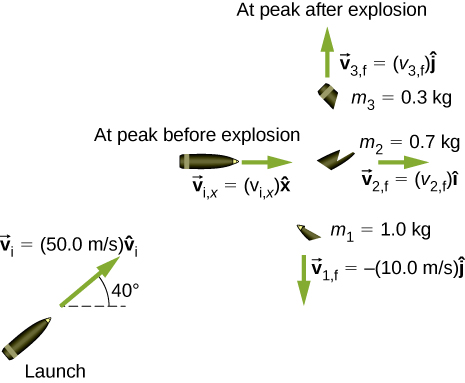1. Find the speeds of the 0.3-kg and 0.7-kg pieces immediately after the break-up.
2. How high from the break-up point does the 0.3-kg piece go before coming to rest?
3. Where does the 0.7-kg piece land relative to where it was ﬁred from?

Two asteroids collide and stick together. The first asteroid has mass of $15\,×\,{10}^{3}\,\text{kg}$ and is initially moving at 770 m/s. The second asteroid has mass of $20\,×\,{10}^{3}\,\text{kg}$ and is moving at 1020 m/s. Their initial velocities made an angle of 20° with respect to each other. What is the final speed and direction with respect to the velocity of the first asteroid?

A 200-kg rocket in deep space moves with a velocity of $(121\,\text{m/s})\hat{i}+(38.0\,\text{m/s})\hat{j}$. Suddenly, it explodes into three pieces, with the first (78 kg) moving at $\text{−}(321\,\text{m/s})\hat{i}+(228\,\text{m/s})\hat{j}$ and the second (56 kg) moving at $(16.0\,\text{m/s})\hat{i}-(88.0\,\text{m/s})\hat{j}$. Find the velocity of the third piece.

A proton traveling at $3.0\,×\,{10}^{6}\,\text{m/s}$ scatters elastically from an initially stationary alpha particle and is deflected at an angle of 85° with respect to its initial velocity. Given that the alpha particle has four times the mass of the proton, what percent of its initial kinetic energy does the proton retain after the collision?

Three 70-kg deer are standing on a flat 200-kg rock that is on an ice-covered pond. A gunshot goes off and the dear scatter, with deer A running at $(15\,\text{m/s})\hat{i}+(5.0\,\text{m/s})\hat{j}$, deer B running at $(-12\,\text{m/s})\hat{i}+(8.0\,\text{m/s})\hat{j}$, and deer C running at $(1.2\,\text{m/s})\hat{i}-(18.0\,\text{m/s})\hat{j}$. What is the velocity of the rock on which they were standing?

A family is skating. The father (75 kg) skates at 8.2 m/s and collides and sticks to the mother (50 kg), who was initially moving at 3.3 m/s and at 45° with respect to the father’s velocity. The pair then collides with their daughter (30 kg), who was stationary, and the three slide off together. What is their final velocity?

An oxygen atom (mass 16 u) moving at 733 m/s at 15.0° with respect to the $\hat{i}$ direction collides and sticks to an oxygen molecule (mass 32 u) moving at 528 m/s at 128° with respect to the $\hat{i}$ direction. The two stick together to form ozone. What is the final velocity of the ozone molecule?

Two cars approach an extremely icy four-way perpendicular intersection. Car A travels northward at 30 m/s and car B is travelling eastward. They collide and stick together, traveling at 28° north of east. What was the initial velocity of car B?